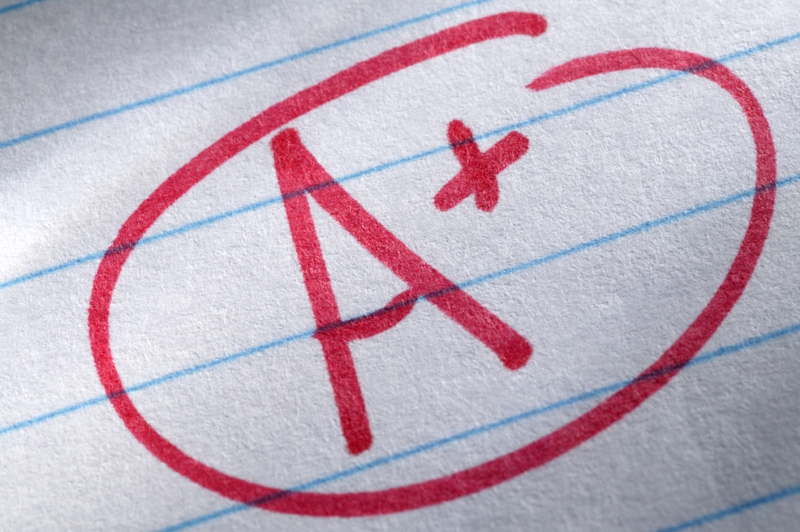Ever take a class where the total class grade is subdivided into percentage for different assignments or tests? For example, the total class grade is calculated by taking a percentage of different categories of work, such as tests, quizzes and homework. Ever wonder how to calculate grade percentage when overall class grades are calculated this way? How does each percentage affect the overall grade? And how to calculate your grade when several grades have a minus sign, such as B-? Here’s how to do it!## How to Calculate Grade Percentage

Many current students, and even recent ones, recall classes where the overall final grade is based on a percentage grade system which is where different assignments or tests are worth a different percentage or “weight” of your overall grade. Generally, common categories include tests, quizzes, class participation, projects and homework. With the table below, we will explain how to calculate grade percentage when a percentage grade system is used.

 Percentage Points Grade Earned Percentage Points Homework 10 90% 9 Tests 40 80% 32 Participation 10 100% 10 Quizzes 20 90% 18 Final Exam 20 80% 16 Your Total Points 85 total percentage points, or 85%

So how did we make this calculation?

1. Look at the total number of percentage points. This will always add up to 100. The numbers in the left column of the above chart represent the total number of percentage points that are possible.

2. Calculate the total number of percentage points that are actually earned. These are the numbers in the right hand column of the above chart. We get these numbers by multiplying the percentage points by the grade. Using the homework example, we multiply 10 by 90%. So you will get 9 earned percentage points.

3. Add up all of the earned percentage points. In the above example, they add up to 85, so the overall grade is an 85%. This is how to calculate grade percentage. If you can get the points in the table above, oh, that is really good.

## How to Convert a Percentage Grade to a Letter Grade

Now that you have gotten your grade percentage, you can compare it to the table below to find out your letter grade, which is more popular nowadays. A majority of top high schools in the United States give grades on a 10 point scale. This means each letter grade represents 10 points. For example, 90-100 is an A grade, 80-89 is a B grade and so on. But how does one calculate their percentage or number grade to a letter grade? Please see the below chart:

 Number or Percentage Grade Letter Grade 93 or above A 90-92 A- 87-89 B+ 83-86 B 80-82 B- 77-79 C+ 73-76 C 70-72 C- 67-69 D+ 63-66 D 60-62 D- 59 or below F

You will note that anything below a 60 is an F (which means failed) while anything above 92 is an A. The remainder of the grade divides the 10 point scale into thirds, so a B- is 80-82, a B is 83-86 and a B+ is 87-89. Letter grades C and D follow the same pattern, as can be seen from the above chart.

ANY IDEAS ABOUT THIS TOPIC?

Please Log In or add your name and email to post the comment.

NAME:
EMAIL: